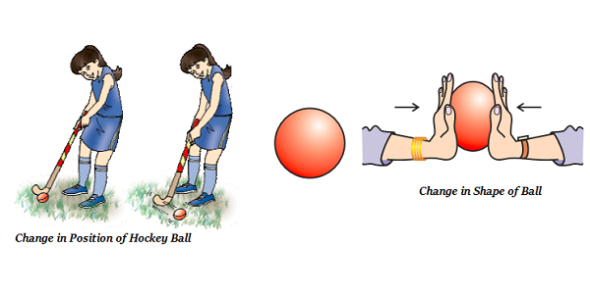# Can You Pass This Physics Quiz On Force?

15 Questions | Attempts: 136SettingsCan you pass this physics quiz on force? Force is the push or pulls on an object that causes it to move from a state of rest. Have you just covered some of the rules and calculations when it comes to forcing? The quiz below is designed to test you on all things on the force. Give it a shot and see if you have what it takes to pass the upcoming exams. All the best!

• 1.
Independent displacement component at each joint of a rigid jointed plane frame are
• A.

Three linear movements

• B.

Two linear movements and one rotation

• C.

One linear movement and two rotations

• D.

Three rotations

• 2.
A single rolling load of 8KN rolls along a girder of 15m span. The absolute maximum bending moment will be
• A.

8 KN-m

• B.

15 KN-m

• C.

30 KN-m

• D.

60 KN-m

• 3.
Deformation of spring produced by a unit load is called
• A.

Stiffness

• B.

Flexibility

• C.

Influence coefficient

• D.

Unit strain

• 4.
By applying the static equations i.e. ΣH = 0, ΣV = 0 and ΣM = 0, to a determinate structure, we may determine
• A.

Supporting reactions only

• B.

Shear forces only

• C.

Bending moments only

• D.

Internal forces only

• E.

All the above.

• 5.
The general expression for the B.M. of a beam of length l is the beam carries
• A.

A uniformly distributed load w/unit length

• B.

A load varying linearly from zero at one end to w at the other end

• C.

An isolated load at mid span

• D.

None of these.

• 6.
Pick up the correct statement from the following:
• A.

For a uniformly distributed load, the shear force varies linearly

• B.

For a uniformly distributed load, B.M. curve is a parabola

• C.

For a load varying linearly, the shear force curve is a parabola

• D.

For a load varying linearly, the B.M. curve is a cubic parabola

• E.

All the above.

• 7.
The effects of shear force and axial force on plastic moment capacity of a structure are respectively to
• A.

Increase and decrease

• B.

Increase and increase

• C.

Decrease and increase

• D.

Decrease and decrease

• 8.
The ratio of moments of inertia of a triangular section about its base and about a centroidal axis parallel to its base, is
• A.

1.0

• B.

1.5

• C.

2.0

• D.

2.5

• E.

3.0

• 9.
Degree of kinematic indeterminacy of a pin-jointed plane frame is given by
• A.

2j-r

• B.

J-2r

• C.

3j-r

• D.

2j+r

• 10.
The ratio of shear stress and shear strain of an elastic material, is
• A.

Modulus of Rigidity

• B.

Shear Modulus

• C.

Young's Modulus

• D.

Modulus of Elasticity

• E.

Both (a) and (b)

• 11.
No. of independent equations to be satisfied for static equilibrium in a space structure
• A.

2

• B.

3

• C.

4

• D.

6

• 12.
A three hinged arch is generally hinged at its supports and
• A.

At one quarter span

• B.

At the crown

• C.

Any where in the rib

• D.

None of these.

• 13.
The forces in the members of simple trusses, may be analysed by
• A.

Graphical method

• B.

Method of joints

• C.

Method of sections

• D.

All the above

• 14.
At yield point of a test piece, the material
• A.

Obeys Hooke's law

• B.

Behaves in an elastic manner

• C.

Regains its original shape on removal of the load

• D.

Undergoes plastic deformation

• 15.
The ratio of lateral strain to axial strain of a homogeneous material, is known
• A.

Yield ratio

• B.

Hooke's ratio

• C.

Poisson's ratio

• D.

Plastic ratio

## Related TopicsBack to top
×

Wait!
Here's an interesting quiz for you.